# Large-Scale Constrained Linear Least-Squares, Solver-Based

This example shows how to recover a blurred image by solving a large-scale bound-constrained linear least-squares optimization problem. The example uses the solver-based approach. For the problem-based approach, see Large-Scale Constrained Linear Least-Squares, Problem-Based.

### The Problem

Here is a photo of people sitting in a car having an interesting license plate.

```load optdeblur [m,n] = size(P); mn = m*n; imshow(P) title(sprintf('Original Image, size %d-by-%d, %d pixels',m,n,mn))```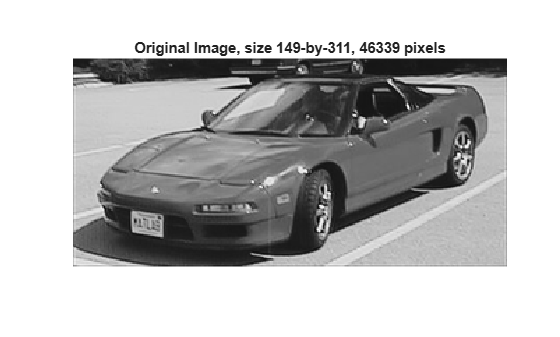The problem is to take a blurred version of this photo and try to deblur it. The starting image is black and white, meaning it consists of pixel values from 0 through 1 in the m x n matrix P.

Simulate the effect of vertical motion blurring by averaging each pixel with the 5 pixels above and below. Construct a sparse matrix `D` to blur with a single matrix multiply.

```blur = 5; mindex = 1:mn; nindex = 1:mn; for i = 1:blur mindex=[mindex i+1:mn 1:mn-i]; nindex=[nindex 1:mn-i i+1:mn]; end D = sparse(mindex,nindex,1/(2*blur+1));```

Draw a picture of D.

```cla axis off ij xs = 31; ys = 15; xlim([0,xs+1]); ylim([0,ys+1]); [ix,iy] = meshgrid(1:(xs-1),1:(ys-1)); l = abs(ix-iy)<=5; text(ix(l),iy(l),'x') text(ix(~l),iy(~l),'0') text(xs*ones(ys,1),1:ys,'...'); text(1:xs,ys*ones(xs,1),'...'); title('Blurring Operator D (x = 1/11)')```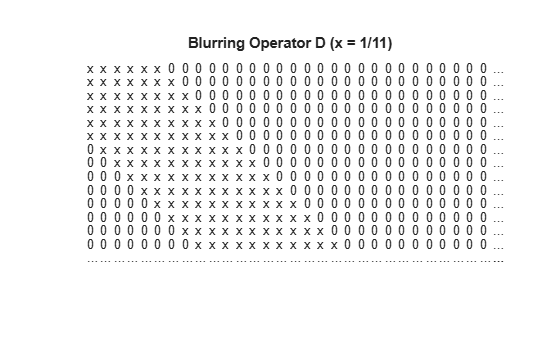Multiply the image P by the matrix D to create a blurred image G.

```G = D*(P(:)); figure imshow(reshape(G,m,n)); title('Blurred Image')```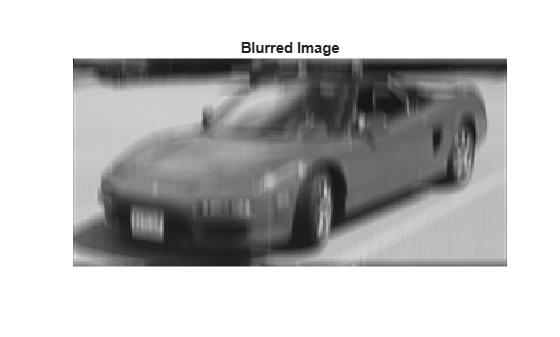The image is much less distinct; you can no longer read the license plate.

### Deblurred Image

To deblur, suppose that you know the blurring operator D. How well can you remove the blur and recover the original image P?

The simplest approach is to solve a least squares problem for x:

$\mathrm{min}\left(‖Dx-G{‖}^{2}\right)$ subject to $0\le x\le 1$.

This problem takes the blurring matrix D as given, and tries to find the x that makes Dx closest to G = DP. In order for the solution to represent sensible pixel values, restrict the solution to be from 0 through 1.

```lb = zeros(mn,1); ub = 1 + lb; sol = lsqlin(D,G,[],[],[],[],lb,ub);```
```Minimum found that satisfies the constraints. Optimization completed because the objective function is non-decreasing in feasible directions, to within the value of the optimality tolerance, and constraints are satisfied to within the value of the constraint tolerance. ```
```xpic = reshape(sol,m,n); figure imshow(xpic) title('Deblurred Image')```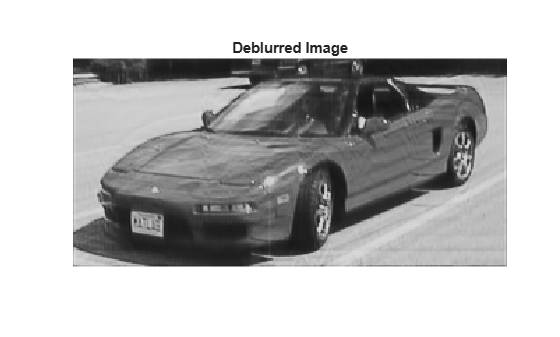The deblurred image is much clearer than the blurred image. You can once again read the license plate. However, the deblurred image has some artifacts, such as horizontal bands in the lower-right pavement region. Perhaps these artifacts can be removed by a regularization.

### Regularization

Regularization is a way to smooth the solution. There are many regularization methods. For a simple approach, add a term to the objective function as follows:

$\mathrm{min}\left(‖\left(D+\epsilon I\right)x-G{‖}^{2}\right)$ subject to $0\le x\le 1$.

The term$\epsilon I$ makes the resulting quadratic problem more stable. Take $\epsilon =0.02$ and solve the problem again.

```addI = speye(mn); sol2 = lsqlin(D+0.02*addI,G,[],[],[],[],lb,ub);```
```Minimum found that satisfies the constraints. Optimization completed because the objective function is non-decreasing in feasible directions, to within the value of the optimality tolerance, and constraints are satisfied to within the value of the constraint tolerance. ```
```xpic2 = reshape(sol2,m,n); figure imshow(xpic2) title('Deblurred Regularized Image')```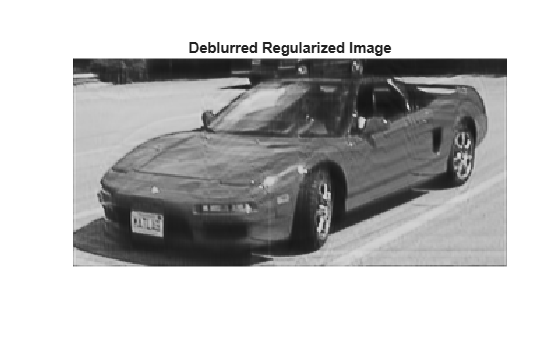Apparently, this simple regularization does not remove the artifacts.